# Pfaffian problem

(diff) ← Older revision | Latest revision (diff) | Newer revision → (diff)

The problem of describing the integral manifolds of maximal dimension for a Pfaffian system of Pfaffian equations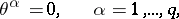(*)

given by a collection ofdifferential-forms which are linearly independent at each point in a certain domain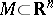(or on a certain manifold). A submanifoldis called an integral manifold of the system (*) if the restrictions of the forms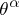toare identically zero. The problem was posed by J. Pfaff (1814).

From a geometric point of view the system (*) determines an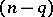-dimensional distribution (a Pfaffian structure) on, that is, a field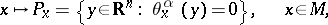of-dimensional subspaces, and the Pfaffian problem consists of describing the submanifolds of maximum possible dimension tangent to this field. The importance of the Pfaffian problem lies in the fact that the integration of an arbitrary partial differential equation can be reduced to a Pfaffian problem. For example, the integration of a first-order equationreduces to the Pfaffian problem for the Pfaffian equation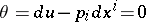on the submanifold (generally speaking with singularities) of the space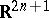defined by the equation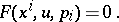A completely-integrable Pfaffian system (and also a single Pfaffian equation of constant class) can be locally reduced to a simple canonical form. In these cases the solution of the Pfaffian problem reduces to the solution of ordinary differential equations. In the general case (in the class of smooth functions) the Pfaffian problem has not yet been solved (1989). The Pfaffian problem was solved by E. Cartan in the analytic case in his theory of systems in involution (cf. Involutional system). The formulation of the basic theorem of Cartan is based on the concept of a regular integral element. A-dimensional subspaceof the tangent spaceis called a-dimensional integral element of the system (*) if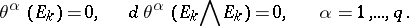The subspaceof the cotangent space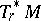generated by the-forms,, where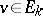andis the operation of interior multiplication (contraction), is called the polar system of the integral element. The integral element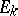is regular if there exists a flag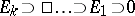for which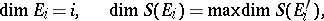where the maximum is taken over all-dimensional integral elementscontaining. Cartan's theorem asserts the following: Letbe a-dimensional integral manifold of a Pfaffian system with analytic coefficients and let, for a certain, the tangent spacebe a regular integral element. Then for any integral element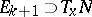of dimension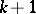there exists in a certain neighbourhood of the pointan integral manifold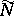, locally containing, for which. Cartan's theorem has been generalized to arbitrary differential systems given by ideals in the algebra of differential forms on a manifold (the Cartan–Kähler theorem).

How to Cite This Entry:
Pfaffian problem. Encyclopedia of Mathematics. URL: http://encyclopediaofmath.org/index.php?title=Pfaffian_problem&oldid=14825
This article was adapted from an original article by D.V. Alekseevskii (originator), which appeared in Encyclopedia of Mathematics - ISBN 1402006098. See original article